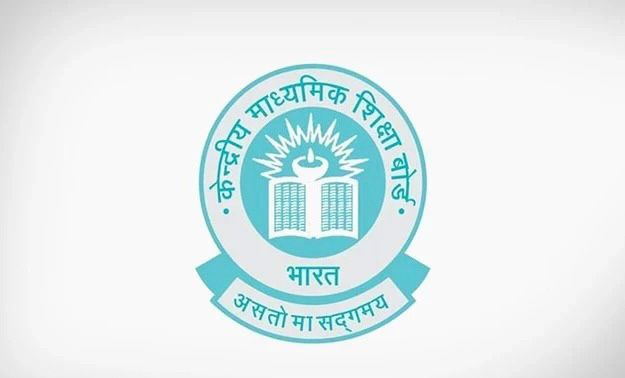# In △ABC and ADEF, ∠F = ∠C, ∠B = ∠E and AB = ½ DE. Then, the two triangles are

CBSE Maths Exam 2021-22 Term 1, question: In △ABC and ADEF, ∠F = ∠C, ∠B = ∠E and AB = ½ DE. Then, the two triangles are## Class 10 Maths Term 1 Exam, options for the question – In △ABC and ADEF, ∠F = ∠C, ∠B = ∠E and AB = ½ DE. Then, the two triangles are

a) Congruent, but not similar

b) Similar, but not congruent

c) Neither congruent nor similar

d) Congruent as well as similar